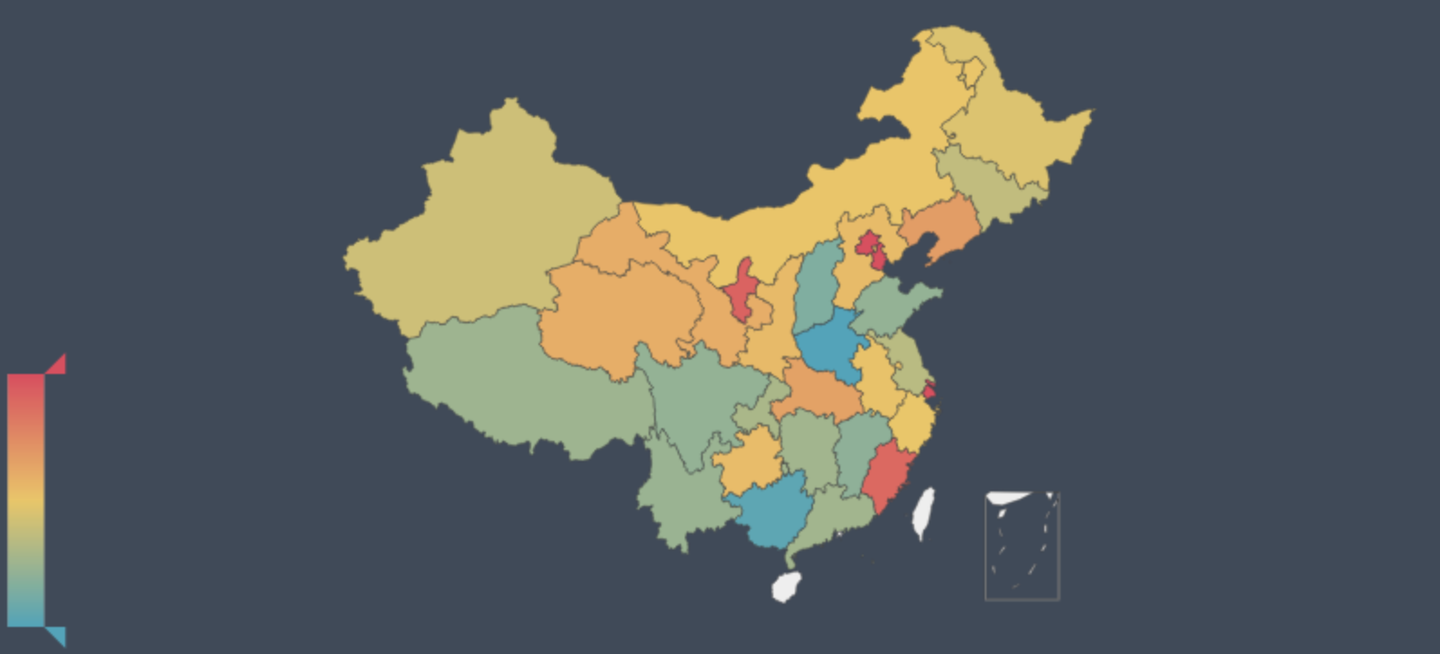``````import numpy as np
from pyecharts import Bar, Line, Overlap

data = [[2017, 940, 700, 74.46],
[2016, 940, 705, 75],
[2015, 942, 700, 74.3],
...
[1977, 570, 27, 5]]

arr = np.array(data)
bar = Bar()
line = Line()
ol = Overlap("历年报考人数与录取率")
ol.render()``````

``````data_born = [[124761, 123626, 122389, ..., 65994],
[14.64, 15.64, 16.57, ..., 24.78]]
arr_born = np.array(data_born)
list_born = list((arr_born*arr_born/1000)[::-1])
bar = Bar("历年报考人数与出生人数")
bar.render()``````

1. 高考人口与出生人数有一定的相关性
2. 适龄人口的高考率逐渐提高

``````from pyecharts import Map

data_prov = [('北京', 6.3), ('重庆', 25), ('上海', 5), ..., ('西藏', 2.53)]
geo = Map("2018各省高考报名人数")
attr, value = geo.cast(data_prov)
geo.add('', attr, value, visual_range=[0, 80], is_visualmap=True, is_map_symbol_show=False)
geo.render()``````

``````data_prov_ad = [('湖南', 11.2), ('江西', 10.4), ('安徽', 14.2), ..., ('浙江', 14)]
geo = Map("2017各省一本录取率")
geo.add("", attr, value, visual_range=[8, 20], is_visualmap=True, is_map_symbol_show=False)
geo.render()``````

（缺海南数据）

════